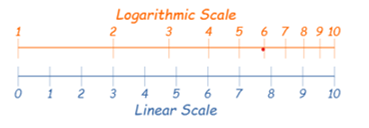A logarithmic scale (or log scale) is a way of displaying numerical data over a very wide range of values in a compact way.

Usually this scale is used for graphs when the largest numbers in the data are hundreds or even thousands of times larger than the smallest numbers.

Such a scale is nonlinear: the numbers 10 and 20, and 60 and 70, are not the same distance apart on a log scale. Rather, the numbers 10 and 100, and 60 and 600 are equally spaced.
Often exponential growth or decay curves are displayed on a log scale.